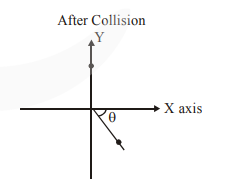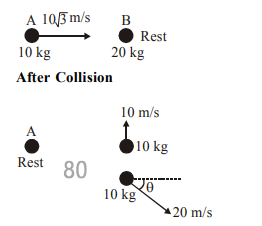# A ball of mass 10 kg moving with a velocity`
Question:

A ball of mass 10 kg moving with a velocity

$10 \sqrt{3} \mathrm{~ms}^{-1}$ along $\mathrm{X}$-axis, hits another ball of mass $20 \mathrm{~kg}$ which is at rest. After collision, the first ball comes to rest and the second one disintegrates into two equal pieces. One of the pieces starts moving along $\mathrm{Y}$-axis at a speed of $10 \mathrm{~m} / \mathrm{s}$. The second piece starts moving at a speed of $20 \mathrm{~m} / \mathrm{s}$ at an angle $\theta$ (degree) with respect to the $\mathrm{X}$-axis.

The configuration of pieces after collision is shown in the figure. The value of T to the nearest integer is _______.Solution:

Before CollisionFrom conservation of momentum along x axis;

$\overrightarrow{\mathrm{P}}_{\mathrm{i}}=\overrightarrow{\mathrm{P}}_{\mathrm{f}}$

$10 \times 10 \sqrt{3}=200 \cos \theta$

$\cos \theta=\frac{\sqrt{3}}{2}$

$\theta=30^{\circ}$